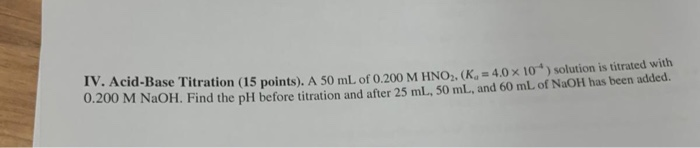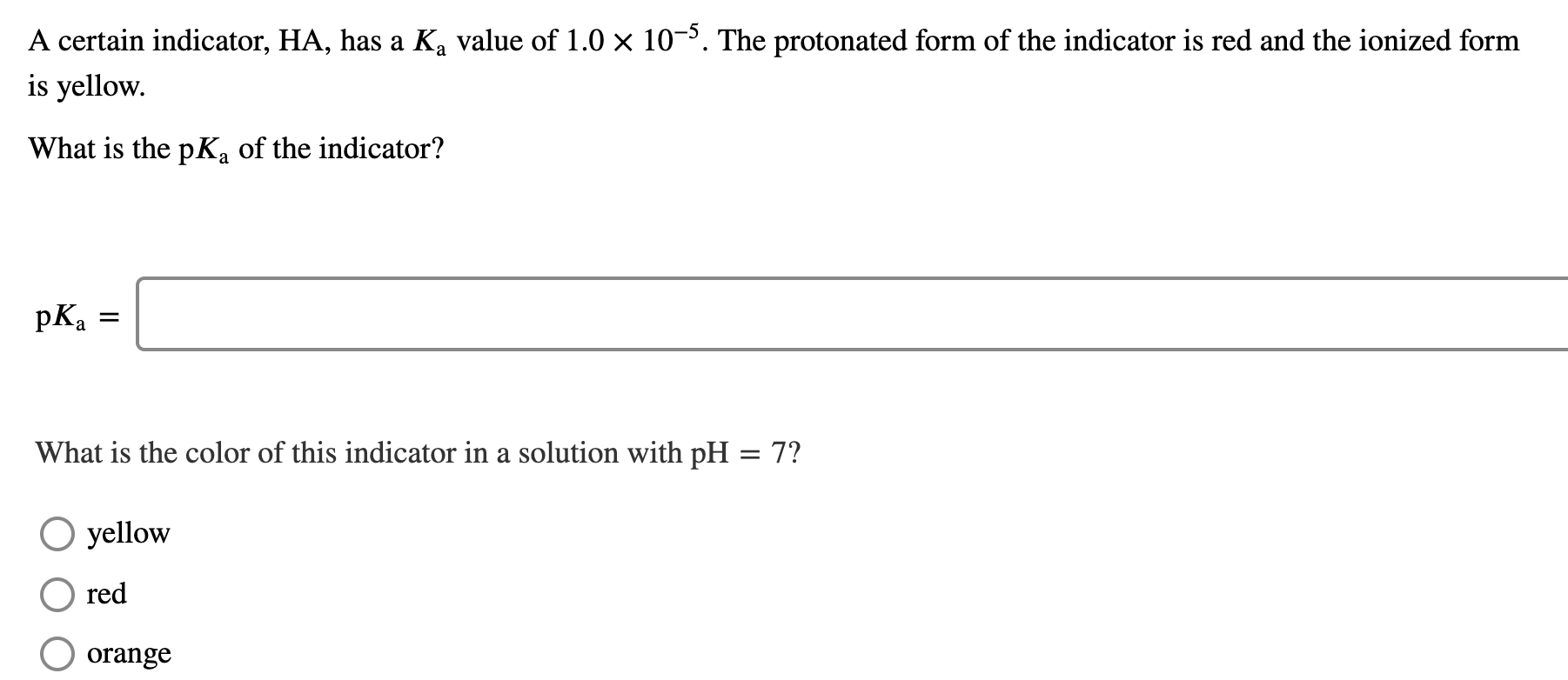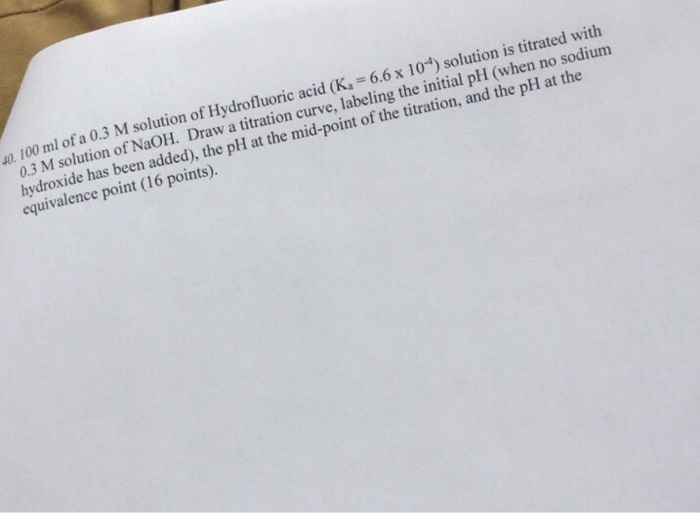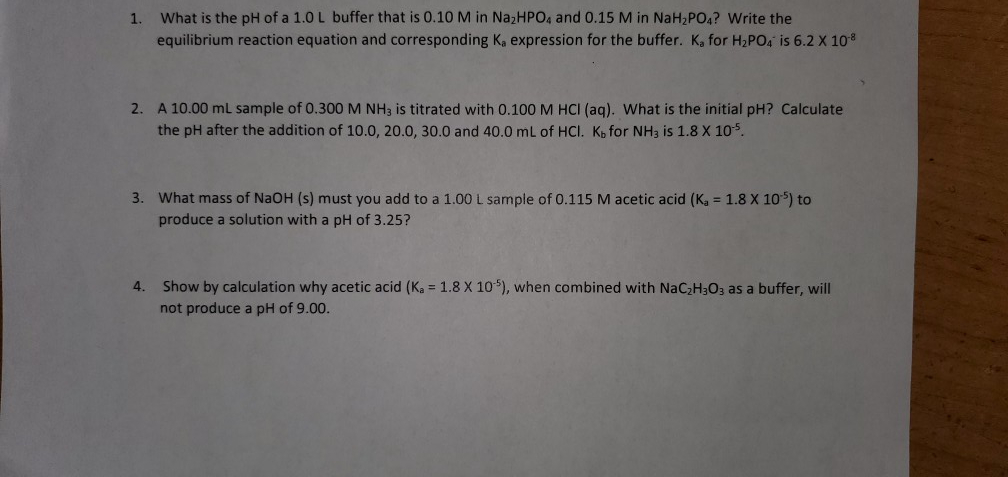Question

# a) An unknown indicator (called “HIn”) has a Ka of 4.0 x 10-6. The color of...

a) An unknown indicator (called “HIn”) has a Ka of 4.0 x 10-6. The color of the neutral form is green, and that of ionized form is red. The indicator is added to a HCl solution, which is then titrated against a NaOH solution. At what pH will the indicator change color?

b) 350. mL of a NaOH solution was added to 500. mL of 2.50 M HNO2. The pH of the mixed solution was 1.75 units greater than that of the original acid solution. Calculate the molarity (in M) of the initial NaOH solution. (Ka of HNO2 is 4.5x10-4)

c) 30.0 mL of 0.100M HCl is titrated with a 0.100 M CH3NH2 solution. Calculate the pH values of the solution at the following titration points (the Ka of CH3NH3+ is noted to be 2.0x10-11): After 30.0 mL of the CH3NH2 solution has been added to the initial acid volume.

a) pKIn of indicator = -log 4.0x10-6 = 5.3979

The reaction is

HIN + OH- ----------------> In- + H2O

The pH of this buffer is

pH = pKIn + log [IN-]/[HIn]

If the ratio [In-][HIN] > 10, the ionisation is almost complete and color changes.

Thus the pH at which color changes = pKIn + 1 = 5.3979+1 = 6.3979

b)

molarity of acid = 2.50 M

pka = -log 4.5x10-4 = 3.347

The pH of acid solution = 1/2[pKa -log C] = 1/2[ 3.347 -log2.50] =2.949

Thus the pH of buffer addition of NaOH = 2.949 + 1.75= 4.699

NaOH + HNO2 ----------------> NaNO2 + H2O

350xa 500x2.50=1250 --- initial mmoles

0 1250-350a 350a --- at equilibrium

the pH of this buffer is given by Hendersen equation as

pH = pKa + log [conjugate base]/[acid]

4.699= 3.347 + log [conjugate base]/[acid]

So  [conjugate base]/[acid]   =22.49  = [350a]/ [1250 -350a]

solving for a , a= 3.419

c)

HCl + CH3NH2 ------------------------> CH3NH3Cl

30x0.1=3 30x0.1=3 0 inital mmoles

0 0 3

[salt ] formed = mmol/volume = 3/60 = 0.05 M

pKb of CH3NH2 = -log 2.0x10-11 = 10.6989

pH of the solution is given as

pH = 1/2[ pKw -pKb - logC] = 1/2[ 14- 10.6989 -log 0.05]

= 2.3010

#### Earn Coins

Coins can be redeemed for fabulous gifts.

Similar Homework Help Questions
• ### An indicator, HIn, has a Ka of 1.5 x 10-6. The color of the neutral form...

An indicator, HIn, has a Ka of 1.5 x 10-6. The color of the neutral form is red and that of the ionized form is yellow. Predict the colour of this indicator in a solution with a pH of 10.50.

• ### in an experiment, 5.00 mL 0.100 M acetic acid (Ka = 1.75 x 10^-5 at 25...

in an experiment, 5.00 mL 0.100 M acetic acid (Ka = 1.75 x 10^-5 at 25 C) was titrated with 0.100 M NaOH solution. The system will attain this pH after 10.0 mL of the titrant has been added

• ### IV. Acid-Base Titration (15 points). A 50 mL of 0.200 M HNO2, (Ka= 4.0 x 10)...IV. Acid-Base Titration (15 points). A 50 mL of 0.200 M HNO2, (Ka= 4.0 x 10) solution is titrated with 0.200 M NaOH. Find the pH before titration and after 25 mL, 50 mL, and 60 mL of NaOH has been added.

• ### 50.0 mL of 0.090 M nitrous acid (HNO2, Ka = 7.1 x 10-4), is titrated with...

50.0 mL of 0.090 M nitrous acid (HNO2, Ka = 7.1 x 10-4), is titrated with 0.100 M NaOH, requiring 45.0 mL of strong base to reach the equivalence point. (a) What will be the pH after 35.0 mL of NaOH have been added? (b) What will be the pH at the equivalence point? (c) What will be the pH after 60.0 mL of NaOH have been added?

• ### pls don't answer if cant help with all :) 1a. 1b. 1c. A certain indicator, HA,...pls don't answer if cant help with all :) 1a. 1b. 1c. A certain indicator, HA, has a Ką value of 1.0 x 10-5. The protonated form of the indicator is red and the ionized form is yellow. What is the pKa of the indicator? pKa What is the color of this indicator in a solution with pH = 7? yellow red O orange The half-equivalence point of a titration occurs half way to the equivalence point, where half of...

• ### A 25.00 mL sample of a 0.250 M aqueous solution CH3NH2 (a weak base) is titrated...

A 25.00 mL sample of a 0.250 M aqueous solution CH3NH2 (a weak base) is titrated with an 0.100 M aqueous solution of HCl (a strong acid.) The molecular and net ionic equation for the reaction is provided below. The Kb value used for CH3NH2 is 4.4x10^-4. Find the pH of the solution after addition of 15.00 mL of the aqueous solution of HCl Molecular: CH3NH2 (aq) + HCl (aq) → CH3NH3+ + Cl— Net ionic:         CH3NH2 (aq) + H+  →...

• ### Of Hydrofluoric acid (Ka-6.6 x 10") solution is titrated with f NaOH. Draw a titration curve, lab...of Hydrofluoric acid (Ka-6.6 x 10") solution is titrated with f NaOH. Draw a titration curve, labeling the initial pH (when no sodium 40. 100 ml ofa 0.3 M olution 0.3 M solution hydroxide has been added), the pH at the mid-point of the titration, and the pH at the equivalence point (16 points). of Hydrofluoric acid (Ka-6.6 x 10") solution is titrated with f NaOH. Draw a titration curve, labeling the initial pH (when no sodium 40. 100 ml...

• ### A 30.0 mL sample of 0.200 M hypochlorous acid (HClO; Ka = 3.0 x 10-8) is...

A 30.0 mL sample of 0.200 M hypochlorous acid (HClO; Ka = 3.0 x 10-8) is titrated with 0.100 M KOH. Calculate the pH after the following volumes have been added 0.0mL 15.0 mL 30.0 mL 45.0 mL 60.0 mL

• ### (1) A weak acid, HA, has a Ka of 6.5 x 10-6 and a concentration of...

(1) A weak acid, HA, has a Ka of 6.5 x 10-6 and a concentration of 0.25 M. 25.5 mL of this weak acid is titrated with 0.10 M NaOH. What is the pH of the solution at the equivalence point? Report your answer with 3 decimal places. (2) The concentration of 16.6 mL of HCl is determined by titrating with NaOH. The titration reaches its endpoint after adding 18.4 mL of 0.829 M NaOH. What is the concentration of...

• ### I need help with #4. "show by calculation why acetic acid (Ka = 1.8 X 10^-5), when combined with NaC2H3O3 as a buffe...I need help with #4. "show by calculation why acetic acid (Ka = 1.8 X 10^-5), when combined with NaC2H3O3 as a buffer, will not produce a pH o 9.00" 1. What is the pH of a 1.0L buffer that is 0.10 M in Naz HPO4 and 0.15 M in NaH2PO4? Write the equilibrium reaction equation and corresponding Ka expression for the buffer. K for H2PO4 is 6.2 X 108 2. A 10.00 mL sample of 0.300 M NH3 is...